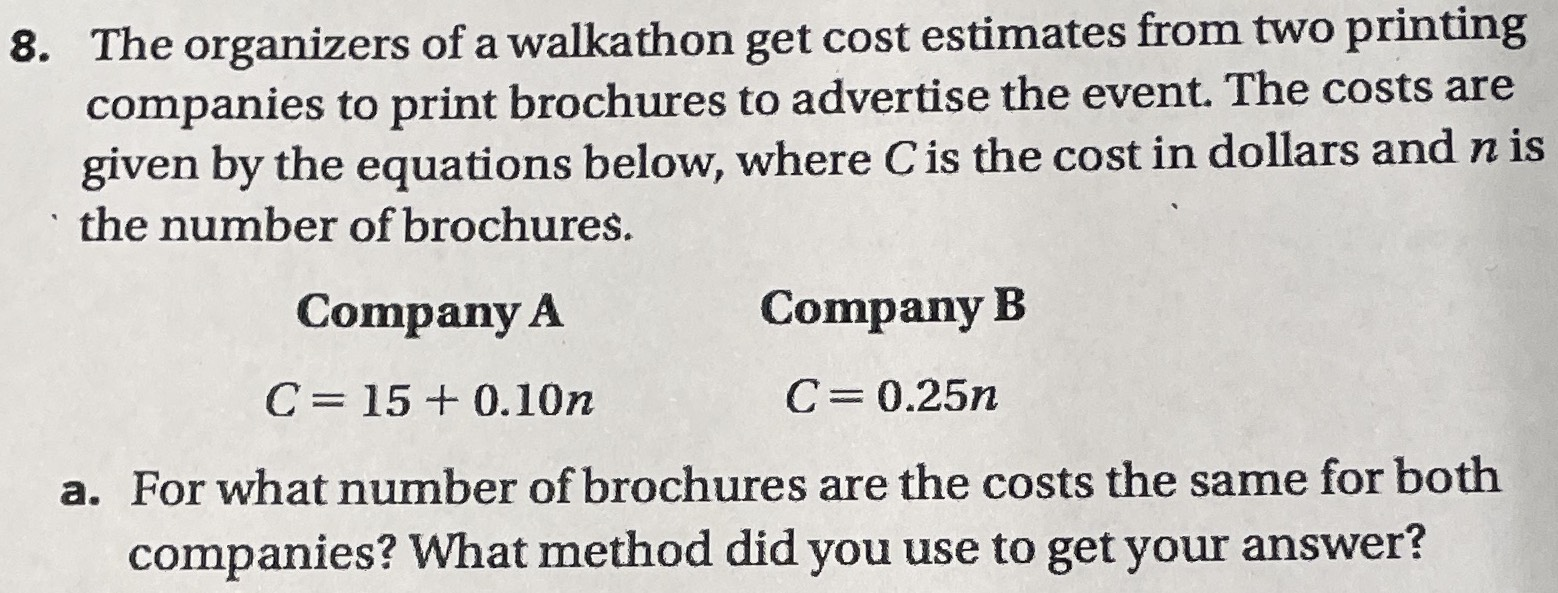### ¿Todavía tienes preguntas de matemáticas?

Pregunte a nuestros tutores expertos
Algebra
Pregunta8. The organizers of a walkathon get cost estimates from two prinumg companies to print brochures to advertise the event. The costs are given by the equations below, where $$C$$ is the cost in dollars and $$n$$ is the number of brochures.

a. For what number of brochures are the costs the same for both companies? What method did you use to get your answer?

$$n = 100$$